# Design IIR Bandpass Filters

In this post, I present a method to design Butterworth IIR bandpass filters.  My previous post  covered lowpass IIR filter design, and provided a Matlab function to design them.  Here, we’ll do the same thing for IIR bandpass filters, with a Matlab function bp_synth.m.  Here is an example function call for a bandpass filter based on a 3rd order lowpass prototype:

N= 3;             % order of prototype LPF
fcenter= 22.5;    % Hz  center frequency, Hz
bw= 5;            % Hz  -3 dB bandwidth, Hz
fs= 100;          % Hz  sample frequency
[b,a]= bp_synth(N,fcenter,bw,fs)
b =  0.0029    0   -0.0087    0    0.0087    0   -0.0029
a =  1.0000   -0.8512    2.6169   -1.3864    2.1258   -0.5584    0.5321


There are seven “b” (numerator) and seven “a” (denominator) coefficients, so H(z) is 6th order.  To find the frequency response:

[h,f]= freqz(b,a,512,fs);
H= 20*log10(abs(h));


The magnitude response of the filter is shown in Figure 1, along with the response of the equivalent analog bandpass filter.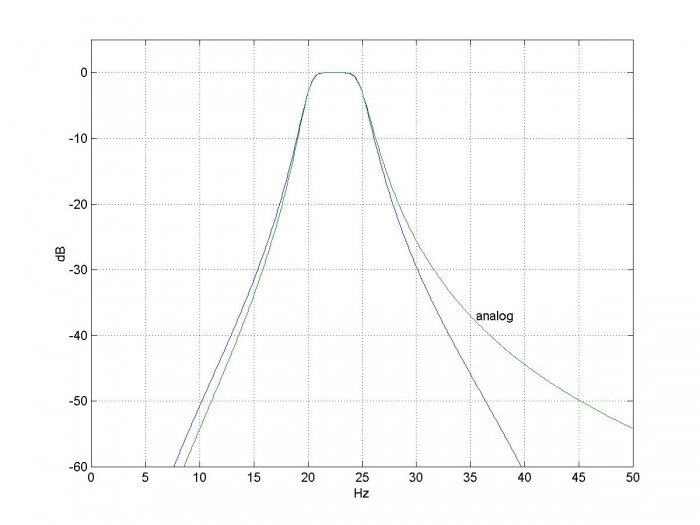Figure 1.  Magnitude response of bandpass filter based on N= 3 lowpass prototype.

fcenter=  22.5 Hz, bw= 5 Hz, and fs= 100 Hz.  The equivalent analog filter’s response is also shown.

## Filter Synthesis

Here is a summary of the steps for computing the bandpass filter coefficients.  Recall from the previous post that F is continuous (analog) frequency in Hz and Ω is continuous radian frequency.

1. Find the poles of a lowpass analog prototype filter with Ωc = 1 rad/s.
2. Given upper and lower -3 dB frequencies of the digital bandpass filter, find the corresponding frequencies of the analog bandpass filter (pre-warping).
3. Transform the analog lowpass poles to analog bandpass poles.
4. Transform the poles from the s-plane to the z-plane, using the bilinear transform.
5. Add N zeros at z= -1 and N zeros at z= +1, where N is the order of the lowpass prototype.
6. Convert poles and zeros to polynomials with coefficients an and bn.

These steps are similar to the lowpass design procedure, with steps 2, 3, 5, and 6 modified for the bandpass case.  Now let’s look at the design procedure in detail.  A Matlab function bp_synth that performs the filter synthesis is provided in Appendix A.

1.  Poles of the analog lowpass prototype filter.  For a Butterworth filter of order N with Ωc = 1 rad/s, the poles are given by [2,3]:

$$p'_{ak}= -sin(\theta)+jcos(\theta)$$

where $$\theta=\frac{(2k-1)\pi}{2N},\quad k=1:N$$

Here we use a prime superscript on p to distinguish the lowpass prototype poles from the yet to be calculated bandpass poles.

2.  Given upper and lower -3 dB frequencies of the digital bandpass filter, find the corresponding frequencies of the analog bandpass filter.  We define fcenter as the center frequency in Hz, and bwHz as the -3 dB bandwidth in Hz.  Then the -3 dB discrete frequencies are :

$$f_1=f_{center}-bw_{Hz}/2$$

$$f_2=f_{center}+bw_{Hz}/2$$

As before, we’ll adjust (pre-warp) the analog frequencies to take the nonlinearity of the bilinear transform into account:

$$F_1=\frac{f_s}{\pi}tan\left(\frac{\pi f_1}{f_s}\right)$$

$$F_2=\frac{f_s}{\pi}tan\left(\frac{\pi f_2}{f_s}\right)$$

We need to define two more quantities:

BWHz = F2 – FHz is the pre-warped -3 dB bandwidth, and

$F_0=\sqrt{F_1F_1}$ is the geometric mean of F1 and F2.

3.  Transform the analog lowpass poles to analog bandpass poles.  See Appendix B for a derivation of the transformation.  For each lowpass pole pa' , we get two bandpass poles:

$$p_a=2\pi F_0\left[\frac{BW_{Hz}}{2F_0}p'_a \pm j\sqrt{1-\left(\frac{BW_{Hz}}{2F_0}p'_a\right)^2}\ \right]$$

4.  Transform the poles from the s-plane to the z-plane, using the bilinear transform.  This is the same as for the IIR lowpass, except there are 2N instead of N poles:

$$p_k=\frac{1+p_{ak}/(2f_s)}{1-p_{ak}/(2f_s)},\quad k=1:2N$$

5.  Add N zeros at z= -1 and N zeros at z= +1.  See Appendix B for details.  We can now write H(z) as:

$$H(z)=K\frac{(z+1)^N(z-1)^N}{(z-p_1)(z-p_2)...(z-p_{2N})}\qquad(1)$$

In bp_synth, we represent the N zeros at -1 and N zeros at +1 as a vector:

     q= [-ones(1,N) ones(1,N)]


6.  Convert poles and zeros to polynomials with coefficients an and bn.  If we expand the numerator and denominator of equation 1 and divide numerator and denominator by z2N, we get polynomials in z-n:

$$H(z)=K\frac{b_0+b_1z^{-1}+...+b_{2N}z^{-2N}}{1+a_1z^{-1}+...+a_{2N}z^{-2N}}$$

The Matlab code to perform the expansion is:

     a= poly(p)
a= real(a)
b= poly(q)


We want H(z) to have a gain of 1 at f = f0.  We can do this by evaluating H(z) at f0 and setting K = 1/|H(f0)|.  The Matlab code is:

     f0= sqrt(f1*f2);
h= freqz(b,a,[f0 f0],fs);
K= 1/abs(h(1));


## Example

Here’s an example IIR bandpass filter based on a 2nd order lowpass.  We’ll show the different poles and zeros computed by bp_synth.m.  (Note the pole and zero values are not printed by the code listed in Appendix A).  The function call is as follows:

N= 2;             % order of prototype LPF
fcenter= 20;      % Hz  center frequency, Hz
bw= 4;            % Hz  -3 dB bandwidth, Hz
fs= 100;          % Hz  sample frequency
[b,a]= bp_synth(N,fcenter,bw,fs)


Here are the pole and zero computed values.  Note Matlab uses an a+bi format for complex numbers.

Two poles of analog lowpass butterworth prototype with Ω’ = 1 rad/s

(p_lp is the same as pa’):

  p_lp =  -0.7071 + 0.7071i  -0.7071 - 0.7071i


Four poles of analog bandpass:

  pa =  1.0e+002 *
-0.1490+ 1.5854i  -0.1234+ 1.3130i  -0.1234- 1.3130i  -0.1490- 1.5854i


Four poles of IIR bandpass:

  p= 0.2053+ 0.8892i   0.3627+ 0.8426i   0.3627- 0.8426i   0.2053- 0.8892i


Two zeros at z= -1 and two zeros at z= 1:

  q =    -1    -1     1     1


The IIR bandpass poles and zeros are shown in Figure 2.  Now let’s look at the filter coefficients.

Numerator coefficients before scaling:

  b =     1     0    -2     0     1


Numerator scale factor:

  K =    0.0134


Filter coefficients b and a:

  b =    0.0134         0   -0.0267         0    0.0134
a =    1.0000   -1.1361    1.9723   -0.9498    0.7009


From K, b, and a we can write the filter’s transfer function:

$$H(z)=.0134*\frac{1-2z^{-2}+z^{-4}}{1-1.1361z^{-1}+1.9723z^{-2}-.9498z^{-3}+.7009z^{-4}}$$

The filter’s magnitude response is plotted as the N=2 curve in Figure 3.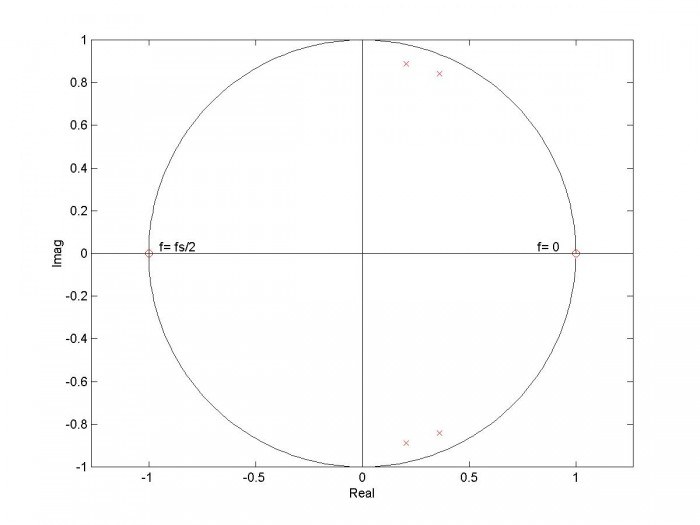Figure 2.  Pole-zero plot of bandpass filter based on 2nd order lowpass prototype.

fcenter= 20 Hz, bw= 4 Hz, and fs= 100 Hz.  Zeros at z= +/-1 are 2nd order.

## Filter Performance

As was the case for lowpass IIR filters, the bandpass IIR response has more rejection than that of the analog prototype as you approach fs/2 (see Figure 1).  This is due to the bilinear transform, which compresses the entire bandwidth into 0 to fs/2.  Thus, the analog zeros at infinity occur at fs/2 for the discrete-time filter.

Figure 3 compares the magnitude response of bandpass filters based on 1st, 2nd, and 3rd order Butterworth lowpass filters.  They all have the same -3 dB bandwidth of 4 Hz.  Figure 4 shows the group delay response of the same filters:  as you would expect for IIR filters, the group delay is not flat.

## Caveats

Performance of IIR filters can be hurt by coefficient quantization and roundoff noise [4,5].  In particular, when the poles are close to the unit circle, quantization of the denominator coefficients can cause a pole to move outside the unit circle, making the filter unstable.  Bandpass filters with narrow bandwidth and higher order are most susceptible.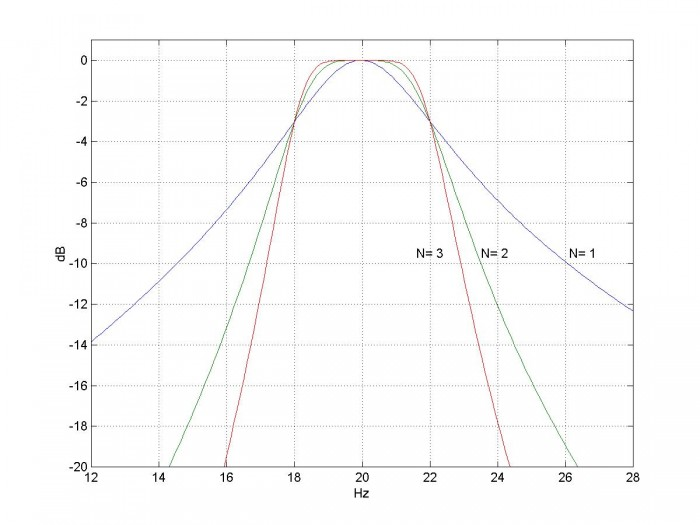Figure 3.  Magnitude response of bandpass filters vs. order of lowpass prototype.

fcenter= 20 Hz, bw= 4 Hz, and fs= 100 Hz.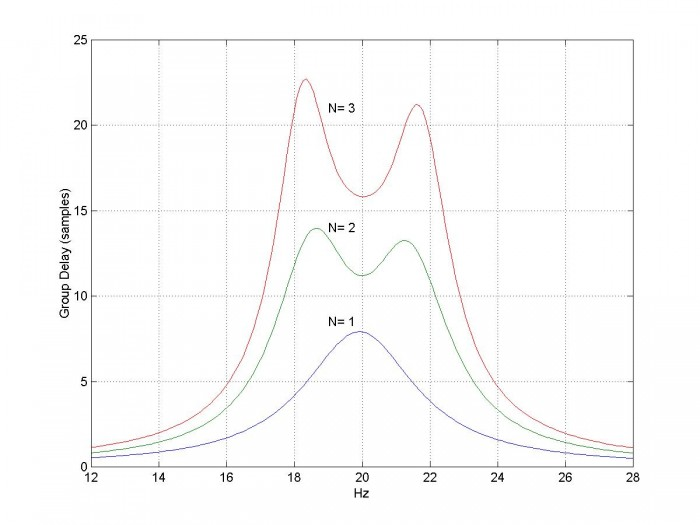Figure 4.  Group Delay of bandpass filters vs. order of lowpass prototype.

fcenter= 20 Hz, bw= 4 Hz, and fs= 100 Hz.  [gd,f]= grpdelay(b,a,512,fs);

## Appendix A.  Matlab Function bp_synth

This program is provided as-is without any guarantees or warranty.  The author is not responsible for any damage or losses of any kind caused by the use or misuse of the program.

%function [b,a]= bp_synth(N,fcenter,bw,fs)      12/29/17 neil robertson
% Synthesize IIR Butterworth Bandpass Filters
%
% N= order of prototype LPF
% fcenter= center frequency, Hz
% bw= -3 dB bandwidth, Hz
% fs= sample frequency, Hz
% [b,a]= vector of filter coefficients
%
function [b,a]= bp_synth(N,fcenter,bw,fs)
f1= fcenter- bw/2;            % Hz lower -3 dB frequency
f2= fcenter+ bw/2;            % Hz upper -3 dB frequency
if f2>=fs/2;
error('fcenter+ bw/2 must be less than fs/2')
end
if f1<=0
error('fcenter- bw/2 must be greater than 0')
end
% find poles of butterworth lpf with Wc = 1 rad/s
k= 1:N;
theta= (2*k -1)*pi/(2*N);
p_lp= -sin(theta) + j*cos(theta);
% pre-warp f0, f1, and f2
F1= fs/pi * tan(pi*f1/fs);
F2= fs/pi * tan(pi*f2/fs);
BW_hz= F2-F1;              % Hz prewarped -3 dB bandwidth
F0= sqrt(F1*F2);           % Hz geometric mean frequency
% transform poles for bpf centered at W0
% note:  alpha and beta are vectors of length N; pa is a vector of length 2N
alpha= BW_hz/F0 * 1/2*p_lp;
beta= sqrt(1- (BW_hz/F0*p_lp/2).^2);
pa= 2*pi*F0*[alpha+j*beta  alpha-j*beta];
% find poles and zeros of digital filter
p= (1 + pa/(2*fs))./(1 - pa/(2*fs));      % bilinear transform
q= [-ones(1,N) ones(1,N)];                % N zeros at z= -1 (f= fs/2) and z= 1 (f = 0)
% convert poles and zeros to numerator and denominator polynomials
a= poly(p);
a= real(a);
b= poly(q);
% scale coeffs so that amplitude is 1.0 at f0
f0= sqrt(f1*f2);
h= freqz(b,a,[f0 f0],fs);
K= 1/abs(h(1));
b= K*b;


## Appendix B.  Lowpass to Bandpass Frequency Transformation

In the s-domain, we want to transform a normalized lowpass filter with -3 dB frequency of 1 rad/s to a bandpass filter with a given bandwidth and center frequency [6,7].  Once we have the bandpass H(s), we can use the bilinear transform to obtain H(z).  Note it is also possible to perform the lowpass to bandpass transformation in the Z-domain .

We define two frequency variables:

Ω = Im(s)             imaginary part of the complex frequency

Ω’ = Im(s’)           imaginary part of the normalized complex frequency

(As in the previous post, we use Ω for continuous frequency and reserve ω for discrete frequency).  To transform H(s’) into H(s), substitute the following for s’:

$$s'=\frac{\Omega_0}{BW}\left(\frac{s}{\Omega_0}+\frac{\Omega_0}{s}\right)\qquad(B-1)$$

where  BW = Ω2-Ω1 = -3 dB bandwidth and $\Omega_0=\sqrt{\Omega_1\Omega_2}$.  If we let s= jΩ, we then have:

$$\Omega'=\frac{\Omega_0}{BW}\left(\frac{\Omega}{\Omega_0}-\frac{\Omega_0}{\Omega}\right)$$

Then, for example, if Ω’= 0, we see that Ω = Ω0.  That is, 0 rad/s transforms to Ω0 rad/s.

Rearranging B-1, we have:

$$s'=\frac{1}{BW}\left(\frac{s^2+\Omega_0^2}{s}\right)$$

Then, solving for s:

$$s=\Omega_0\left[\frac{BW}{2\Omega_0}s' \pm j\sqrt{1-\left(\frac{BW}{2\Omega_0}s'\right)^2}\ \right]$$

If we replace s’ with a normalized lowpass pole location pa’, we have:

$$p_a=\Omega_0\left[\frac{BW}{2\Omega_0}p'_a \pm j\sqrt{1-\left(\frac{BW}{2\Omega_0}p'_a\right)^2}\ \right]\qquad(B-2)$$

For each lowpass pole pa’ , we get two bandpass poles.  Thus if the lowpass filter has order N, H(s) for the bandpass filter has order 2N.  The IIR filter’s poles are computed from pa using the bilinear transform, as discussed in step 4 in the section on filter synthesis.

For the Matlab function, we replace Ω0 with 2πF0 and replace BW with 2π*BWHz:

$$p_a=2\pi F_0\left[\frac{BW_{Hz}}{2F_0}p'_a \pm j\sqrt{1-\left(\frac{BW_{Hz}}{2F_0}p'_a\right)^2}\ \right]$$

Figures B.1 and B.2 plot the s-plane poles computed by equation B-2 for lowpass order N= 2 and N=4, respectively.  The zeros at s= 0 will be discussed next.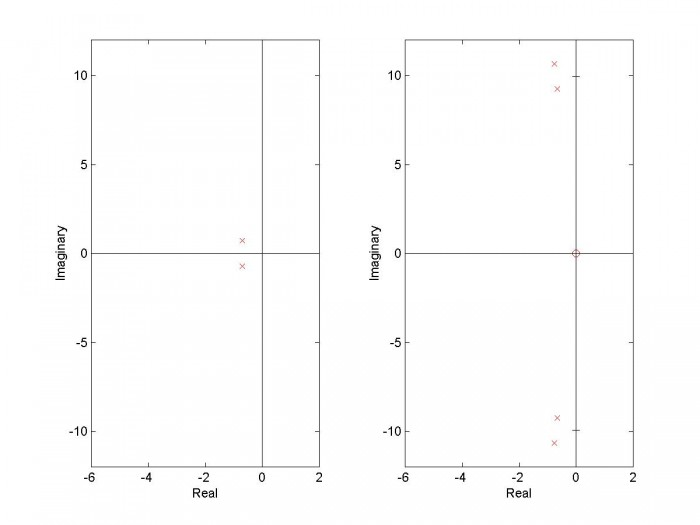Figure B.1  s-plane transformation of N= 2 lowpass poles to bandpass poles and zeros.

Left:  Normalized lowpass Complex-conjugate poles, N= 2

Right:  Bandpass poles and zero for Ω0= 10 rad/s and BW= 2 rad/s

The zero at s= 0 is 2nd order.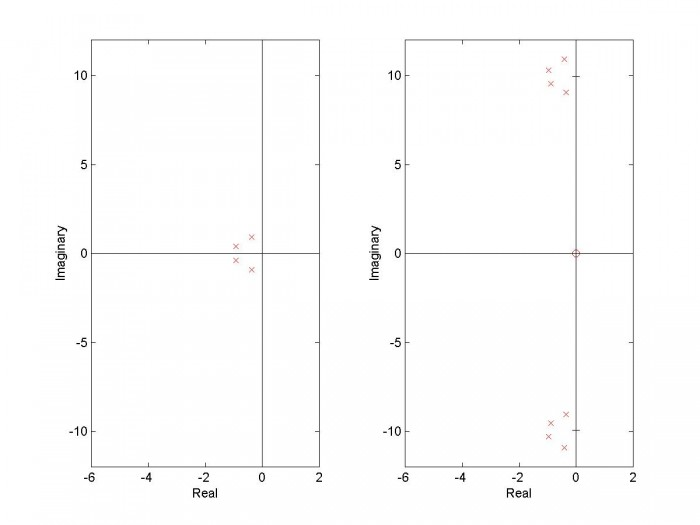Figure B.2  s-plane transformation of N= 4 lowpass poles to bandpass poles and zeros.

Left:  Normalized lowpass Complex-conjugate poles, N= 4

Right:  Bandpass poles and zeros for Ω0= 10 rad/s and BW= 2 rad/s

The zero at s= 0 is 4th order.

## Zeros of H(s) and H(z)

Consider a single-pole normalized lowpass transfer function in s’:

$$H(s')=\frac{1}{s'-\alpha}$$

It has a zero at s’= infinity.  From B-1, we have for the corresponding bandpass filter:

$$H(s)=\frac{1} {\frac{\Omega_0}{BW}\left(\frac{s}{\Omega_0}+\frac{\Omega_0}{s}\right)-\alpha}$$

H(s) has a zero at s= 0 and a zero at infinity.  In general, if H(s’) has N zeros at infinity, then H(s) has N zeros at 0 and N zeros at infinity.

In my last post , I showed that the bilinear transform maps a zero at infinity to one at fs/2.  Thus z = exp(j2πf/fs) = exp(jπ) = -1.  For a zero at s= 0, z= exp(j0)= 1.  We can now write H(z) in pole-zero format as:

$$H(z)=K\frac{(z+1)^N(z-1)^N}{(z-p_1)(z-p_2)...(z-p_{2N})}$$

The numerator of H(z) can be written as $(z^2-1)^N$.  If you expand this, you get the numerator polynomial coefficients as follows:

     N= 1:  b= [1 0 -1]
N= 2:  b= [1 0 -2 0 1]
N= 3:  b= [1 0 -3 0 3 0 -1]


Thus odd-power numerator coefficients are always zero and all numerator coefficients are integers before scaling by K.

Figure B.3 shows the z-plane pole-zero plot of a bandpass filter based on a 4th order Butterworth lowpass.  You can compare it to the pole-zero plot of the continuous-time bandpass filter in Figure B.2.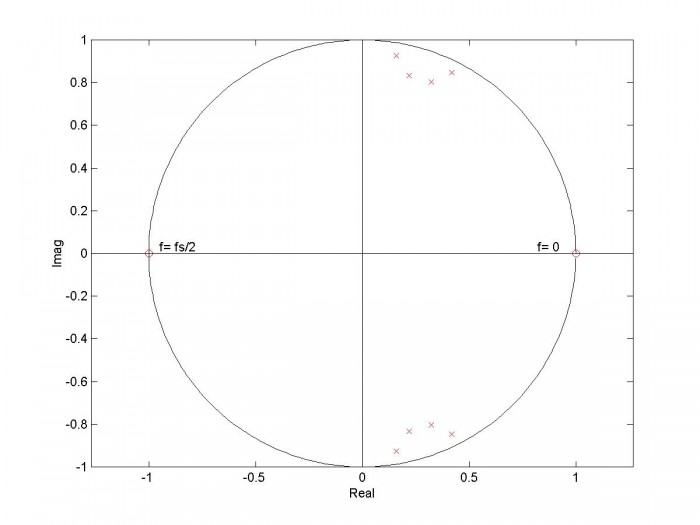Figure B.3.  Z-plane pole-zero plot of bandpass filter based on 4th order lowpass prototype.

fcenter= 20 Hz, bw= 5 Hz, and fs= 100 Hz.  Zeros at z= +/-1 are 4th order.

## Appendix C  Notation

In this article, we added to the notation used in the post on lowpass filter synthesis .

Notation for normalized lowpass continuous frequencies:

normalized complex frequency                 s’= σ + jΩ’

Notation for bandpass filter continuous frequencies:

-3 dB frequencies                                       Ω1, Ω2  rad/s

geometric mean frequency                        $\Omega_0=\sqrt{\Omega_1\Omega_2}$   rad/s

- 3 dB bandwidth, Hz                                   BWHz  Hz

geometric mean frequency, Hz                   F0  Hz

Notation for bandpass filter discrete frequencies:

-3 dB frequencies, Hz                                 f1, f2  Hz

geometric mean frequency, Hz                  f0  Hz

The notation already developed in the previous post:

continuous frequency                                  F  Hz

complex frequency                                      s = σ + jΩ

discrete frequency                                       f  Hz

discrete normalized radian frequency         ω = 2πf/fsradians, where fs = sample frequency

Finally, the Matlab function represents -3 dB discrete bandwidth (Hz) as bw and the -3 dB continuous bandwidth (Hz) as BW_hz.

## References

1.  Robertson, Neil , “Design IIR Butterworth Filters Using 12 Lines of Code”, Dec 2017 https://www.dsprelated.com/showarticle/1119.php

2.  Williams, Arthur B. and Taylor, Fred J., Electronic Filter Design Handbook, 3rd Ed., McGraw-Hill, 1995, section 2.3

3.  Analog Devices Mini Tutorial MT-224, 2012 http://www.analog.com/media/en/training-seminars/tutorials/MT-224.pdf

4.  Oppenheim, Alan V. and Shafer, Ronald W., Discrete-Time Signal Processing, Prentice Hall, 1989, sections 6.8 and 6.9.

5.   Lyons, Richard G., Understanding Digital Signal Processing, 2nd Ed., Pearson, 2004, section 6.7

6.  Blinchikoff, Herman J., and Zverev,Anatol I., Filtering in the Time and Frequency Domains, Wiley, 1976, section 4.4.

7.  Nagendra Krishnapura , “E4215: Analog Filter Synthesis and Design Frequency Transformation”,  4 Mar. 2003  http://www.ee.iitm.ac.in/~nagendra/E4215/2003/handouts/freq_transformation.pdf

8.  Oppenheim, Alan V. and Shafer, Ronald W., Discrete-Time Signal Processing, Prentice Hall, 1989, section 7.2

Neil Robertson                  January, 2018        revised 4/3/2019

[ - ]
Comment by October 12, 2018Could you please give me any clue how to calculate K? The problem is I'm implementing the algorithm in Scala language therefore I do not have library which implements freqz function.

[ - ]
Comment by October 12, 2018I think I've found - K = 1/|H(f0)|

[ - ]
Comment by October 12, 2018Sashko,

That's correct!

regards,

Neil

[ - ]
Comment by January 8, 2018Thanks Neil,

Another buried secret of Matlab unearthed. I tested N = 4 and got same as matlab butter

[b,a]= butter(4,2*[.1-.1/2,.1+.1/2]);

[b,a]= bp_synth(4,.1,.1,1);

Kaz

[ - ]
Comment by January 8, 2018Kaz,

Thanks, I had not tested it vs the Matlab function.  Nice to know it matches.

Neil

[ - ]
Comment by April 18, 2018Hi,

Nice to see the 'behind the scenes' equations! Thanks!

This could be interesting to other readers: another way to implement bandpass filter is to use the 'sum-of-allpass' structure, which provides orthogonal control over the 'Q' and 'f0' from each coefficient.

H = 0.5*(1-A(z)), where A(z) is a 2nd order allpass filter. By choosing the lattice structure for A(z), you get orthogonal control over the filter specifications.

For example:

%some settings
fs = 48e3;
%some bandpass filter specs
f0 = 10e3;
bw = 1e3;
%design using butterworth
[b,a] = butter(1,[f0-bw f0+bw]/(fs/2),'bandpass');
Hbutter = filt(b,a);

%                 0.1163 - 0.1163 z^-2
% Hbutter =   -----------------------------
%             1 - 0.4614 z^-1 + 0.7673 z^-2

%design using sum-of-allpass
k1 = -cos(2*pi*f0/fs);
k2 = (1-tan(2*pi*bw/fs)) / (1+tan(2*pi*bw/fs));
Az = filt([k2 k1*(1+k2) 1],[1 k1*(1+k2) k2]);
Hbp = (1/2)*(1-Az);

%            0.1163 - 0.1163 z^-2
% Hbp =   ----------------------------
%          1 - 0.4574 z^-1 + 0.7673 z^-2

%Show they are the same
fvtool(Hbp.num{1}, Hbp.den{1}, b,a,'Fs', fs);

You can see that k1 only depends on f0, and k2 only depends on the bandwidth. This allows for easy tuning. For example, if you keep k1 constant and sweep k2 and vice versa, you get those responses:Those sum-of-allpass structures are also extremely robust to coefficient and data quantization, less prone to limit cycles and have many other nice properties.

More details, examples and cool things that one can do with allpass filters can be found here:

The Digital All-Pass Filter: A Versatile Signal Processing Building Block

[ - ]
Comment by April 18, 2018Thanks -- looks interesting.

Neil

[ - ]
Comment by November 2, 2018One small correction for Step 3: "For each lowpass pole pa' , we get two complex-conjugate bandpass poles" - actually, we get a complex conjugate pair of band-pass poles only from a real low-pass pole. But we always get two complex conjugate pairs of band-pass poles from a complex conjugate pair of low-pass poles pa' and ~pa' .

[ - ]
Comment by April 2, 2019Hi Igorinov,

I think my previous reply to you was not quite correct.  I agree completely with your statement.  I updated the text of the post and pdf file.

regards,

Neil

[ - ]
Comment by April 14, 2023I'm trying to figure out how to convert this to use with a Chebyshev2 filter. Would it just be the lowpass filter line? I'm aware a percentage of ripple would need adding here, too.

[ - ]
Comment by April 14, 2023Lee,

I have not looked at designing type 2 Chebyshev filters.  Such filters would have different zeros as well as poles from the Butterworth filter.  I have not looked at Elliptic filters, either.

regards,

Neil

To post reply to a comment, click on the 'reply' button attached to each comment. To post a new comment (not a reply to a comment) check out the 'Write a Comment' tab at the top of the comments.